﻿ Dynamics of <img src=image/pra3.png></img> Kinks by Using Adomian Decomposition Method

### Dynamics of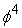Kinks by Using Adomian Decomposition Method

Arash Ghahraman

American Journal of Numerical Analysis

## Dynamics ofKinks by Using Adomian Decomposition Method

Arash GhahramanDepartment of Physics, Faculty of Basic Science, Chabahar Maritime University, Chabahar, Iran

### Abstract

This paper studies nonlinear waves in the presence of weak external perturbation. Dynamical behavior of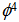kink is examined. Adomian decomposition method is employed to study the kink-impurity interaction. As a result, an analytical approximate solution is derived. Then some of the first terms of the series solution are considered to show the kink behavior in the presence of impurity.

• Arash Ghahraman. Dynamics of Kinks by Using Adomian Decomposition Method. American Journal of Numerical Analysis. Vol. 4, No. 1, 2016, pp 8-10. http://pubs.sciepub.com/ajna/4/1/2
• Ghahraman, Arash. "Dynamics of Kinks by Using Adomian Decomposition Method." American Journal of Numerical Analysis 4.1 (2016): 8-10.
• Ghahraman, A. (2016). Dynamics of Kinks by Using Adomian Decomposition Method. American Journal of Numerical Analysis, 4(1), 8-10.
• Ghahraman, Arash. "Dynamics of Kinks by Using Adomian Decomposition Method." American Journal of Numerical Analysis 4, no. 1 (2016): 8-10.

 Import into BibTeX Import into EndNote Import into RefMan Import into RefWorks

1
Prev Next

### 1. Introduction

Nonlinear problems are of interest to physicists and other scientists because most systems are inherently nonlinear in nature. It is well known that investigation of the wave propagation through inhomogeneous and disordered media plays an important role in the study of nonlinear physical phenomena. To gain a deeper understanding of the behavior of nonlinear excitations in disordered systems, one has to investigate the interactions of soliton with separate localized inhomogeneities (impurities) . An external potential can be added to the equation of motion as perturbative terms . The interaction of solitary waves with spatial inhomogeneous is of considerable importance for physical applications. Impurities and/or defects are present even in the purest of material samples and their effect on the motion of solitary waves must be considered when the dynamics of such solutions are important in the problem at hand [2, 3].

As is well-known, when waves scatter on a potential they can be partly reflected and partly transmitted. For the fields of soliton solutions the situation is more complicated as soliton can’t split and thus must either bounce, pass through or become trapped inside the potential. This dynamics is very sensitive to the value of all the parameters of the model and to the initial conditions as well [1, 4].

In this paper we study Adomian Decomposition Method (ADM) [5, 6, 7, 8] for investigating the effect of impurity on the motion of solitary-wave solutions on the nonlinearequation. We illustrate the method by examining he motion of soliton solution in the presence of Delta function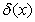as an impurity .

### 2.Theory

The model we consider istheory in (1+1) dimensions. This model is a nonlinear, Lorentz invariant, scalar field theory which is not integrable. In this paper an analytical model for the interaction offield with an external potential is presented. The general form of the action in an arbitrary metric is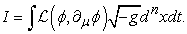(1)

Where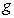is the determinant of the metric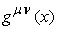. Energy density of the field-potential can be found by varying both the field and the metric [1, 3, 4]. For the Lagrangian of the form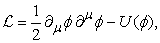(2)

With the potential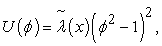(3)

where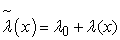. This potential parameter has a constant value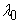in the region where the soliton is located and far away from the position of the soliton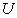is zero as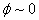. After varying the action, the equation of motion is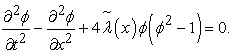(4)

The kink solutions of the non-perturbativeequation is defined by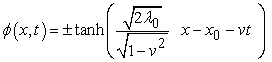(5)

In this paper ADM is used as approximate analytical method for solving nonlinear equation (4). It is known that the Adomian decomposition method is a powerful and effective method for solving a wide class of linear and nonlinear differential (ODEs and PDEs) and integral equations [3, 8, 9]. The Adomian decomposition method was first proposed by G. Adomian in 1980. In general, applying the ADM (and its improvements), we construct an approximate analytic solution as an infinite series, which may converge to an exact solution. We consider exact solutions and truncated series solutions for numerical calculations, visualizations, and comparisons. For the nonlinear PDEs, the idea of the Adomian decomposition method consists in decomposition of the unknown function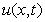of an equation into an infinite series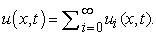(6)

Where the component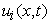are determined recursively. The nonlinear term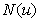is represented as an infinite series of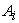which is called Adomian polynomials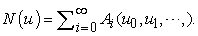(7)

There exist several schemes for calculating Adomian polynomials. Here the following scheme considered for calculation of Adomian polynomials for the nonlinear term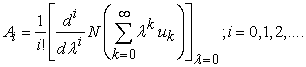(8)

In an operator form equation (4) becomes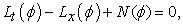(9)

where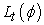,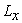and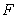are defined by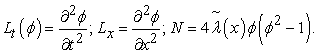(10)

The inverse operator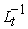is defined by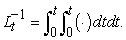(11)

Applyingto both sides of equation (9) and using initial conditions we obtain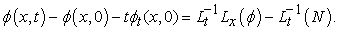(12)

Substituting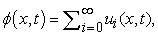(13)

and the nonlinear term by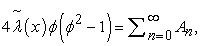(14)

into equation (12) gives the recursive relations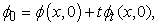(15)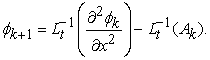(16)

The initial conditions are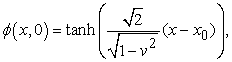(17)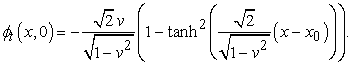(18)

Figure 1 shows single soliton solution at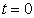. The first few components are given by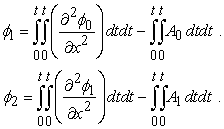(19)

Figure 2, and Figure 3 show soliton – impurity interaction with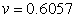and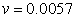respectively.Download asVeiw figureFigures index
Figure 1. single soliton solution at μ(x,0)Download asVeiw figureFigures index
Figure 2. Soilton-impurity interaction with v=0.6057, at (a)t=0.68. (b) t=80. (c)t=2.50. (d)t=10.0. (e)t=20. (f) t=25Download asVeiw figureFigures index
Figure 3. Soilton-impurity interaction with v=0.0057, at (a) t=0.30. (b) t=0.45. (c) t=0.80. (d)t=1.25. (e)t=3.0. (f) t=5.0

### 4. Conclusion

We have studied thekink scattering by an impurity. Adomian decomposition method (ADM) employed directly in the treatment of this model to drive a series of approximations to the solution. The Dirac Delta function was considered as an impurity and kink–impurity interaction was investigated analytically. The analytic approximation to the solution provide us the ability to study physical properties of the problem easily. The computational program with MAPLE enable us to have a series term easily and there is no need to do that much computations.

### References

  A. Ghahraman and K. Javidan, “Analytical Formulation for phi4 Field Potential Dynamics,” Braz J Phys, 2011.In article  M. B. Fogel, S. Trullinger, A. R. Bishop and J. A. Krumhansl, “Dynamics of sine-Gordon soliton in the pesence of perturbation,” physical review B, vol. 15, no. 3, pp. 1578-1592, 1977.In article  “Interaction of noncommutative solitons with defects,” BRAZILIAN JOURNAL OF PHYSICS, vol. 38, no. 4, pp. 610-614, 2008.In article View Article  A. Ghahraman and S. Eskandari, “A New Model for Dynamics of Sine-Gordon solitons in The Presence of Perturbation,” Advances in Natural and Applied Sciences, vol. 8, no. 15, pp. 21-24, 2014.In article  J. Biazar and R. Islam, “Solution of wave equation by Adomian decomposition method and the restrictions of the method,” Applied Mathematics and Computation, no. 149, p. 807-814, 2004.In article View Article  J. Biazar and M. Pourabd, “A Maple Program for Solving Systems of Linear and Nonlinear Integral Equations by Adomian Decomposition Method,” INTERNATIONAL JOURNAL OF COMPUTERS FOR MATHEMATICAL LEARNING, vol. 2, no. 29, pp. 1425-1432, 2007.In article  H. Bulut and B. H. M., “Geometrical Interpretation and A Comparative Study Between Three Different Methods For Solving The Non-Linear Kdv Equation,” Journal of Advanced Research in Scientific Computing, vol. 2, no. 1, pp. 69-76, 2010.In article  H.M.Baskonus, H.Bulut and Y.Pandir, “The natural transform decomposition method for linear and nonlinear partial differantial equations,” Mathematics In Engineering, Science And Aerospace (MESA), vol. 5, no. 1, pp. 111-126, 2014.In article  H. Bulut, F.B.M.Belgacem and H.M.Baskonus, “Partial fractional differential equation systems solutions by Adomian decomposition method implementation,” in 4th International Conference On Mathematical and Computational Applications, Manisa, Turkey, 11-13 June 2013.In article  T. M. R. Filho and A. Figueiredo, “a Maple package for the symmetry analysis of differential equations,” Computer Physics Communications, no. 182, p. 467-476, 2011.In article View Article  M. Younis, S. T. R. Rizvi and S. Ali, “Analytical and soliton solutions: Nonlinear model of nanobioelectronics transmission lines,” Applied Mathematics and Computation, no. 265, p. 994-1002, 2015.In article View Article